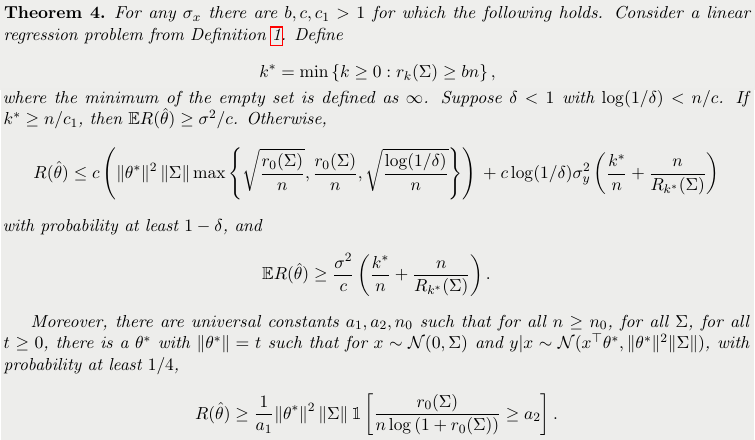# Benign Overfitting in Linear Regression

##### Posted on Oct 11, 20210 Comments

Consider when a perfect fit to training data in linear regression is compatible with accurate prediction.

The paper gives a characterization of linear regression problems for which the minimum norm interpolating prediction rule has near-optimal prediction accuracy

• the characterization is in terms of two notions of the effective rank of the data covariance
• overparameterization is essential for benign overfitting in the setting that the number of directions in parameter space that are unimportant for prediction must significantly exceed the sample size

The paper considers quadratic loss and linear prediction rules, and assume that the dimension of the parameter space is large enough that a perfect fit is guaranteed. The question is to ask when it is possible to fit the data exactly and still compete with the prediction accuracy.

There might be many interpolating solutions since more parameters than the sample size, choose the parameter vector with the smallest norm among all vectors that give perfect predictions on the training sample.

The main result is a finite sample characterization of when overfitting is benign in this setting. The linear regression problem depends on the optimal parameter $\theta^\star$ (ideal value of the parameters corresponding to the linear prediction rule that minimizes the expected quadratic loss) and the covariance $\Sigma$ of the covariate $x$.

There is a classical decomposition of the excess prediction error into two terms,• the first is rather standard: provided that the scale of the problem (the eigenvalues of $\Sigma$) is small compared to the sample size $n$, the contribution to $\hat\theta$ can view as coming from $\theta^\star$ is not too distorted.
• the second term is more interesting since it reflects the impact of the noise in the labels on prediction accuracy. This part is small if and only if the effective rank of $\Sigma$ in the subspace corresponding to low variance directions is large compared to $n$. this can be viewed as a property of significant overparameterization: fitting the training data exactly but with near-optimal prediction accuracy occurs iff there are many low variance (and hence unimportant) directions in parameter space where the label noise can be hidden.

Published in categories Note